Next: 7.5 Complete OpAmp A709 Up: 7. Simulation Results Previous: 7.3 Five-Stage CML Ring

# 7.4 Thermal Analysis of an Emitter-Coupled Pair

Matching considerations are extremely important for emitter-coupled pairs and current mirrors. A schematic of a typical circuit is shown in Fig. 7.19. Even though this circuit is topologically very similar to the CML inverter in Fig. 6.12 its numerical behavior is quite different due to the large dynamic range of the node voltages. Especially when considering thermal coupling between the transistors, the problem becomes extremely nasty as the transistors are very sensitive to the thermal boundary condition.

To aid convergence when simulating thermally fully coupled transistors a special iteration scheme was used. In the first block the thermal quantities were ignored until an electrical solution was found. In the second block, the lattice temperature was added to the solution vector without considering the coupling effects caused by the node temperatures. This was also found to be advantageous when stepping through the DC transfer curve hence this block was also used for the consecutive steps. However, as the condition of the transient problem is much better, this block is not used for transient simulation. Only after a proper temperature distribution inside the devices has been established for the new voltage boundary conditions, can the complete equation system be used. In terms of the IPL this iteration scheme reads

ThermalDD
{
Electric : ~Iterate.Blocks.DDBlock
{
ignoreQuantities = "LatticeTemperature,NodeTemperature";
enter            = ~Extern.firstStep;
}
ThermalPre : ~Iterate.Blocks.DDBlock
{
ignoreQuantities = "NodeTemperature";
enter            = ~Extern.firstStep || !~Solve.transient;
}
Thermal : ~Iterate.Blocks.DDBlock;
}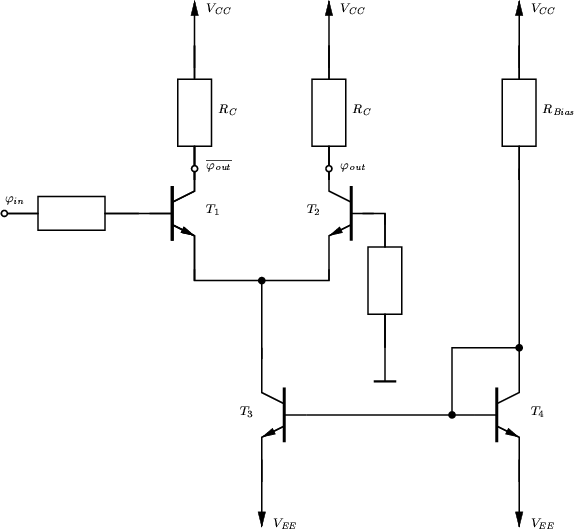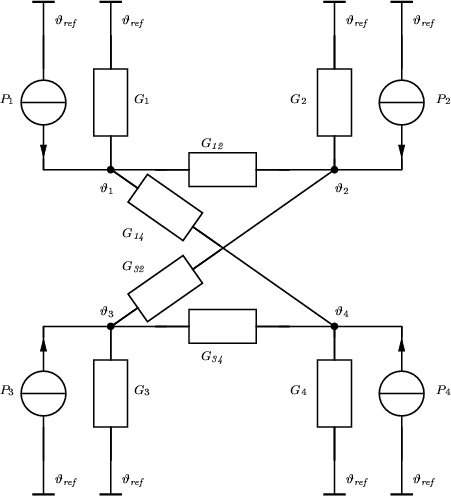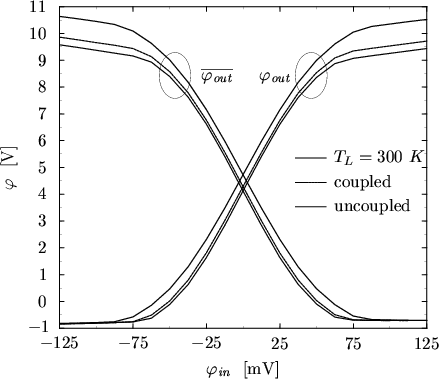When the transistors are not thermally coupled their characteristics will not match. This is due to the fact, that one of the transistors conducts more current than the other which results in different power consumption and hence different self-heating. To model the thermal coupling of the transistors the thermal circuit shown in Fig. 7.20 has been assumed. The power dissipated by the transistors Ti is given by Pi and a thermal case-ambient conductivity Gi = 6 mW/K has been assumed. In addition, T1 is assumed to be thermally coupled with T2 and T3 with T4, respectively, using G12 = G34 = 2 mW/K. Asymmetrical thermal coupling has been assumed with coupling conductivities G14 = 1.8I>mW/K and G32 = 0.2 mW/K [45,42].

The simulated DC transfer characteristic using three different thermal models is shown in Fig. 7.21. For the first model a constant lattice temperature TL = 300 K was assumed. The second model considered self-heating of the transistors and case-ambient conductivity Gi but no thermal coupling between the transistors. Finally, the third model assumes thermal coupling between some of the transistors as stated above. The contact temperatures of the transistors are shown in Fig. 7.22 and Fig. 7.23, respectively. For self-heating without coupling the collector currents are overestimated and correspondingly the available output voltage underestimated due to the large voltage drops at RC. When neglecting self-heating effects, the collector currents are too low.

A similar behavior can be observed for transient simulation. The response of the circuit to a sinusoidal input signal with amplitude 0.1 V and f = 5 MHz is shown in Fig. 7.24. Although the thermal time constant is sufficiently small compared to the input signal frequency, the contact temperatures stay approximately constant over time because the circuit operates in small-signal mode.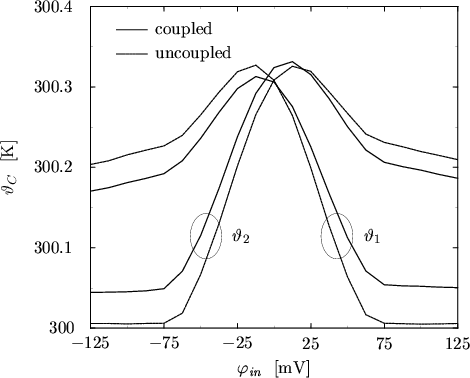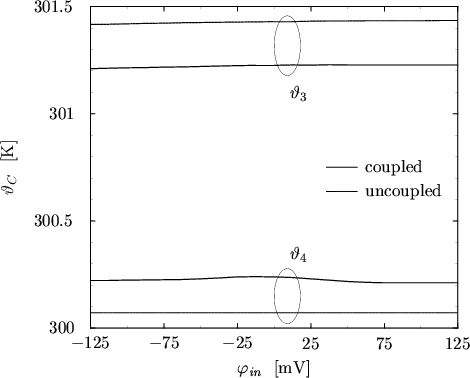Next: 7.5 Complete OpAmp A709 Up: 7. Simulation Results Previous: 7.3 Five-Stage CML Ring
Tibor Grasser
1999-05-31